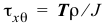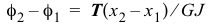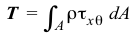# Torsion of Circular Shafts

In the questions below, select the correct answer.

Name Email
1. Torsional shear strain varies linearly across a homogeneous cross section.
2. Torsional shear strain is a maximum at the outermost radius for a homogeneous and a nonhomogeneous cross section.
3. Torsional shear stress is a maximum at the outermost radius for a homogeneous and a nonhomogeneous cross section.
4. The formulacan be used to find the shear stress on a cross section of a tapered shaft.
5. The formulacan be used to find the relative rotation of a segment of a tapered shaft.
6. The formulacan be used to find the shear stress on a cross section of a shaft subjected to distributed torques.
7. The formulacan be used to find the relative rotation of a segment of a shaft subjected to distributed torques.
8. The equationcannot be used for nonlinear materials.
9. The equationcan be used for a nonhomogeneous cross section.
10. Internal torques jump by the value of the concentrated external torque at a section.